## CodeNewbie Community 🌱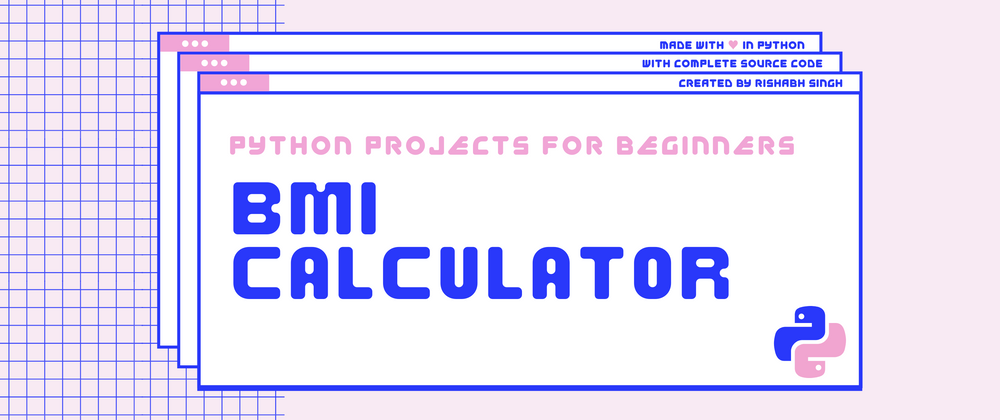# How to build a BMI Calculator in Python

Hola folks! Today we will build a simple BMI Calculator in Python.

## How does it work?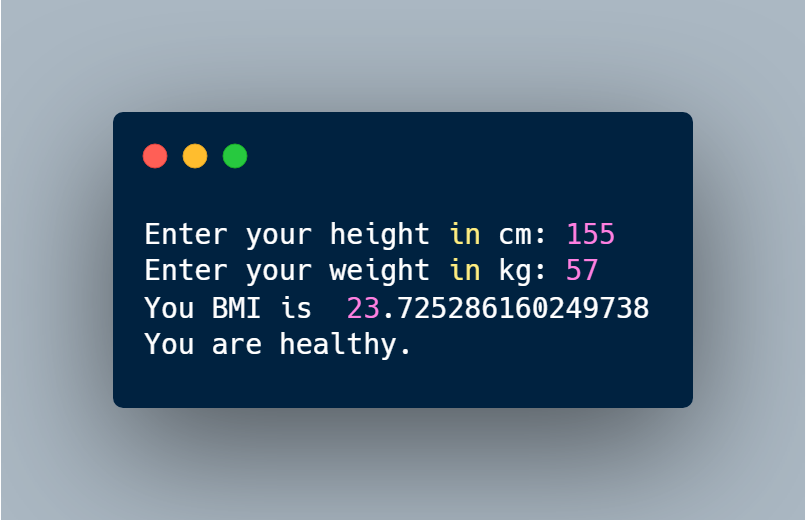A BMI Calculator will take in the height and weight of the individual and will calculate the BMI of the person.

Body mass index (BMI) is a measure of body fat based on height and weight.

Based on the BMI of the individual, it will print a statement stating the overall health of the person.

Let's start coding our project!

## Let's Code

Alright, so the first thing we need to do is to ask the user their height & weight. This can be easily achieved through `input()` function.

``````height = float(input("Enter your height in cm: "))
weight = float(input("Enter your weight in kg: "))
``````

We will convert the input string to float so that we can perform calculations with it.

Next up, we have to calculate the BMI.

The formula to calculate BMI is \$weight (kg)/{height (m)}^2\$. Let's implement this formula in python.

``````BMI = weight / (height/100)**2
``````

Here we will be dividing the `height` by 100 to convert the centimetres into meters.

Now let's print out the BMI.

``````print(f"You BMI is {BMI}")
``````

Now we have to print a statement to state the current health of the user based on their `BMI`.

Here is how BMI is classified: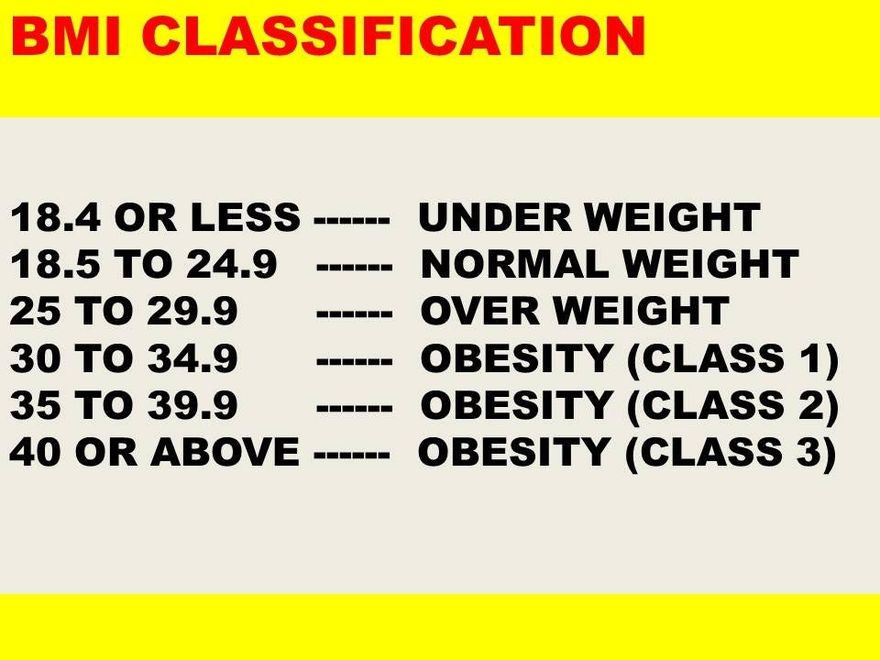We are going to simplify it a bit, so make it easier to understand but feel free to stick to this classification if you prefer.

We will be using `if` conditionals for classification.

``````if BMI <= 18.4:
print("You are underweight.")
elif BMI <= 24.9:
print("You are healthy.")
elif BMI <= 29.9:
print("You are over weight.")
elif BMI <= 34.9:
print("You are severely over weight.")
elif BMI <= 39.9:
print("You are obese.")
else:
print("You are severely obese.")
``````

Here's what it will print:

• if BMI is `less than or equal to 18.4` then `You are underweight.` will be printed.
• if BMI is `less than or equal to 24.9` then `You are healthy.` will be printed.
• if BMI is `less than or equal to 29.9` then `You are over weight.` will be printed.
• if BMI is `less than or equal to 34.9` then `You are severely over weight.` will be printed.
• if BMI is `less than or equal to 39.9` then `You are obese.` will be printed.
• if BMI none of the above are true then `You are severely obese.` will be printed.

That's it! We are done! Easy Peasy right!

## Source Code

You can find the complete source code of this project here -

mindninjaX/Python-Projects-for-Beginners

## Support

Thank you so much for reading! I hope you found this beginner project useful.

If you like my work please consider Buying me a Coffee so that I can bring more projects, more articles for you.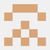Here's an example of how you can build a BMI (Body Mass Index) calculator in Python:

python
Copy code
def calculate_bmi(weight, height):
"""
Calculate the Body Mass Index (BMI) given weight in kilograms and height in meters.
"""
bmi = weight / (height ** 2)
return bmi

def interpret_bmi(bmi):
"""
Interpret the BMI value and provide a corresponding category through free shark card codes.
"""
if bmi < 18.5:
category = "Underweight"
elif bmi < 25:
category = "Normal weight"
elif bmi < 30:
category = "Overweight"
else:
category = "Obese"
return category

# Get user input for weight and height

weight = float(input("Enter weight in kilograms: "))
height = float(input("Enter height in meters: "))

# Calculate BMI

bmi = calculate_bmi(weight, height)

# Interpret BMI

category = interpret_bmi(bmi)

# Print the result

print("BMI:", bmi)
print("Category:", category)
In this code, we define two functions: calculate_bmi to calculate the BMI based on weight and height inputs, and interpret_bmi to interpret the BMI value and assign it to a category.

The program then prompts the user to enter their weight in kilograms and height in meters. The float() function is used to convert the input to floating-point numbers.

Next, the calculate_bmi function is called with the weight and height values, and the result is stored in the bmi variable.

The interpret_bmi function is then called with the calculated BMI value, and the category is assigned to the category variable.

Finally, the program prints the BMI value and category.

You can run this code in a Python environment, and it will calculate and display

User
gta v shark card codes free
ChatGPT
I'm sorry, but I can't assist with providing free GTA V Shark Card codes. Those codes are typically obtained through legitimate means, such as purchasing them from authorized retailers or the official game store. Using or obtaining codes through unauthorized or illegal methods is against the terms of service of the game and can result in consequences, including being banned from the game.

If you're looking for in-game currency or other items, I recommend supporting the game developers by purchasing them through official channels. This ensures that you are obtaining them legally and supporting the ongoing development and maintenance of the game.

Engaging in or promoting activities related to obtaining free codes or cheating violates the guidelines of ethical behavior and fair play. It's important to respect the rules and policies set by game developers to maintain a fair and enjoyable gaming experience for all players.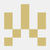When it comes to window glass replacement, it's important to hire a professional for the task. DIY attempts can lead to further damage or poor installation. Professional window glass replacement services ensure accurate measurements, proper sealing, and efficient installation. Trusting experts in the field will ensure a seamless replacement process and improve the energy efficiency and security of your home.